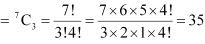# Class 11 Maths NCERT Solutions for Chapter 7 Permutations and Combinations Exercise 7.4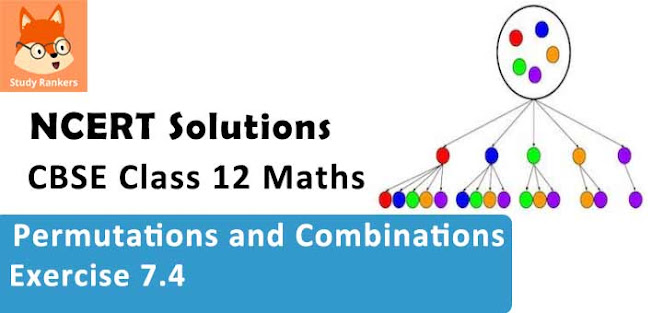### Permutations and Combinations Exercise 7.4 Solutions

1. If nC8 = nC2, find nC2.

Solution

It is known that, nCa = nCb ⇒ a = b or n = a+ b
Therefore,
nC8 = nC2 ⇒ n = 8 + 2 = 10
∴ nC2 = 10C2 =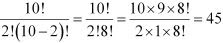2. Determine n if
(i) 2nC3 = nC3 = 12 : 1
(ii) 2nC3 = nC3 = 11 : 1

Solution

(i)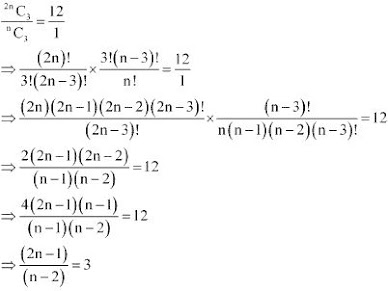⇒ 2n - 1 = 3(n - 2)
⇒ 2n - 1 = 3n - 6
⇒ 3n - 2n = -1 + 6
⇒ n = 5

(ii)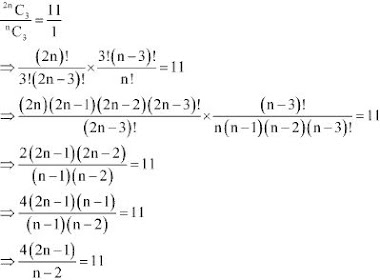⇒ 4(2n - 1) = 11(n - 2)
⇒ 8n - 4 = 11n - 22
⇒ 11n - 8n = -4 + 22
⇒ 3n = 18
⇒ n = 6

3. How many chords can be drawn through 21 points on a circle?

Solution

For drawing one chord on a circle, only 2 points are required.
To know the number of chords that can be drawn through the given 21 points on a circle, the number of combinations have to be counted.
Therefore, there will be as many chords as there are combinations of 21 points taken 2 at a time.
Thus, required number of chords = 21C2 = 21!/2!(21 -2)! = 21!/2!19! = (21×20)/2 = 210

4. In how many ways can a team of 3 boys and 3 girls be selected from 5 boys and 4 girls?

Solution

A team of 3 boys and 3 girls is to be selected from 5 boys and 4 girls.
3 boys can be selected from 5 boys in  5C3  ways .
3 girls can be selected from 4 girls in 4C3  ways.
Therefore, by multiplication principle, number of ways in which a team of 3 boys and  3 girls can be selected = 5C3 × 4C3  = (5!/3!2!) × (4!/3!1!)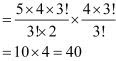5. Find the number of ways of selecting 9 balls from 6 red balls, 5 white balls and 5 blue balls if each selection consists of 3 balls of each colour.

Solution

There are a total of 6 red balls, 5 white balls, and 5 blue balls.
9 balls have to be selected in such a way that each selection consists of 3 balls of each colour.
Here,
3 balls can be selected from 6 red balls in 6C3 ways.
3 balls can be selected from 5 white balls in 5C3 ways.
3 balls can be selected from 5 blue balls in 5C3 ways.
Thus, by multiplication principle, required number of ways of selecting 9 balls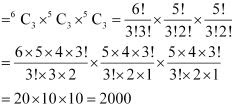6. Determine the number of 5 card combinations out of a deck of 52 cards if there is exactly one ace in each combination.

Solution

In a deck of 52 cards, there are 4 aces. A combination of 5 cards have to be made in which there is exactly one ace.
Then, one ace can be selected in 4C1 ways and the remaining 4 cards can be selected out of the 48 cards in 48C4 ways.
Thus, by multiplication principle, required number of 5 card combinations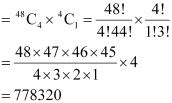7. In how many ways can one select a cricket team of eleven from 17 players in which only 5 players can bowl if each cricket team of 11 must include exactly 4 bowlers?

Solution

Out of 17 players, 5 players are bowlers.
A cricket team of 11 players is to be selected in such a way that there are exactly 4 bowlers.
4 bowlers can be selected in 5C4 ways and the remaining 7 players can be selected out of the 12 players in  12C7 ways.
Thus, by multiplication principle, required number of ways of selecting cricket team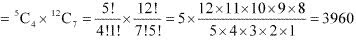8. A bag contains 5 black and 6 red balls. Determine the number of ways in which 2 black and 3 red balls can be selected.

Solution

There are 5 black and 6 red balls in the bag.
2 black balls can be selected out of 5 black balls in 5C2 ways and 3 red balls can be selected out of 6 red balls in 6C3 ways.
Thus, by multiplication principle, required number of ways of selecting 2 black and 3 red balls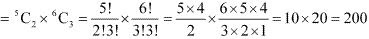9. In how many ways can a student choose a programme of 5 courses if 9 courses are available and 2 specific courses are compulsory for every student?

Solution

There are 9 courses available out of which, 2 specific courses are compulsory for every student.
Therefore, every student has to choose 3 courses out of the remaining 7 courses. This can be chosen in 7C3 ways.
Thus, required number of ways of choosing the programme